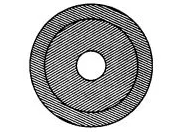# An archery target has three regions formed

Question:

An archery target has three regions formed by three concentric circles as shown in figure. If the diameters of the concentric circles are in the ratio 1:2:3,

then find the ratio of the areas of three regions.Solution:

Let the diameters of concentric circles be k, 2k and 3k.

$\therefore$ Radius of concentric circles are $\frac{k}{2}, k$ and $\frac{3 k}{2}$.

$\therefore \quad$ Area of inner circle, $A_{1}=\pi\left(\frac{k}{2}\right)^{2}=\frac{k^{2} \pi}{4}$

$\therefore$ Area of middle region, $A_{2}=\pi(k)^{2}-\frac{k^{2} \pi}{4}=\frac{3 k^{2} \pi}{4}$

$\left[\because\right.$ area of ring $=\pi\left(R^{2}-r^{2}\right)$, where $R$ is radius of outer ring and $r$ is radius of inner ring]

and area of outer region, $A_{3}=\pi\left(\frac{3 k}{2}\right)^{2}-\pi k^{2}$

$=\frac{9 \pi k^{2}}{4}-\pi k^{2}=\frac{5 \pi k^{2}}{4}$

$\therefore \quad$ Required ratio $=A_{1}: A_{2}: A_{3}$

$=\frac{k^{2} \pi}{4}: \frac{3 k^{2} \pi}{4}: \frac{5 \pi k^{2}}{4}=1: 3: 5$